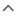• A
• B
• C
• D
• F
• G
• H
• I
• J
• K
• L
• M
• N
• O
• P
• R
• S
• T
• V
• A
• B
• C
• D
• F
• G
• H
• I
• J
• K
• L
• M
• N
• O
• P
• R
• S
• T
• V## Tesla Price List

Tesla Cars List Price
Tesla Model Y Price
RM 199,000 - 288,000
Tesla Model 3 Price Not On Sale
Tesla Model S Price Not On Sale
Tesla Model X Price Not On Sale
• ### How much is a Tesla car in Malaysia?The cheapest Tesla car price is the Tesla Model Y RM 199,000 and the highest price is the Tesla Model Y RM 288,000
• ### How many models does Tesla have?Tesla has 4 models and 3 variants for you to choose from.
• ### Does Tesla have an SUV model?Tesla SUV Cars: Tesla Model Y